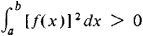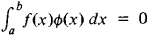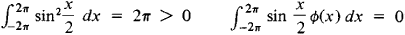# Complete System of Functions

The following article is from The Great Soviet Encyclopedia (1979). It might be outdated or ideologically biased.

## Complete System of Functions

A system of functions Φ = {ɸ(x)} defined on the interval [a, b] is said to be complete if there exists no function f(x) such thatand such that f(x) is orthogonal to all the functions ɸ(x) of Φ —in other words, such thatfor any ɸ(x) of ɸ. The integrals here are Lebesgue integrals.

A system may be complete on one interval and not complete on another. For example, 1, sin x, cos x, …, sin nx, cos nx, … form a complete system of functions on the interval [0, 2π]. They do not, however, form a complete system on the interval [– 2π, 2π] becausefor each ɸ (x) of the system under consideration. A system of square integrable functions will be complete if, and only if, any square integrable function on the interval [a, b] can be approximated in the mean to any desired degree of accuracy by a linear combination of functions from the system.